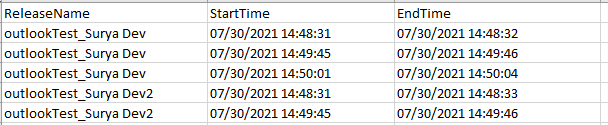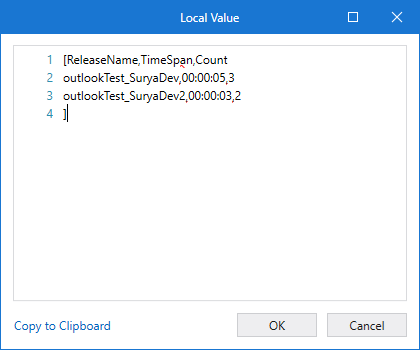# Data table - club Release name column and also calculate time

Hi. Hope you are doing good.

I’ve an input data table as follows:I need to prepare an output data table by grouping release names and also calculate two more columns as follows:

I’m thinking that I should use database select, groupby queries but not sure how to do this. Can someone please help me to create that output data table?

1 Like

Tried to create linq but it’s only calculating seconds, needs some modification.
Check once and let me know how exactly you need data in Total Time.

(From r In dtInput.AsEnumerable
Group r By k = r(“ReleaseName”).tostring Into grp = Group
Let sumstart = grp.Sum(Function (x) x.Field(Of Date)(“StartTime”).TimeOfDay.TotalSeconds)
Let sumend = grp.Sum(Function (x) x.Field(Of Date)(“EndTime”).TimeOfDay.TotalSeconds)
Let subtract = CInt(sumstart) - CInt(sumend)
Let ra = New Object() {grp(0)(“ReleaseName”),subtract,grp.Count}
test.xaml (7.3 KB)

4 Likes

@ermanoj3101

I want total time as a timespan HH:mm:ss. That too endtime-starttime should be calculated for every row in the group and then these results should be added.

just replace subtract with` TimeSpan.FromSeconds(subtract)`

1 Like

@kumar.varun2

I’ve one more doubt of this substraction. I feel that @ermanoj3101 query adds all start-times in a group and adds all end-times in a group and then calculates difference of these sums

I need to calculate end time-start time for each row in the group and then sum these values.

``````(From row In inputDT
Group row By rn = row("ReleaseName").ToString
Into grp = Group
Let dur = grp.Sum(Function (x) DateTime.Parse(x("EndTime").ToString).TimeOfDay.TotalSeconds -DateTime.Parse(x("StartTime").ToString).TimeOfDay.TotalSeconds)
Let ra = New Object() {grp(0)("ReleaseName"),TimeSpan.FromSeconds(dur),grp.Count}
``````Groupby TimeSpan Count.xaml (7.5 KB)

1 Like

I thought that’s what you want, anyways you can check @kumar.varun2 query.

Thanks.

1 Like

Thank you @kumar.varun2 . I’ll try this

Thanks @kumar.varun2 . I used your linq, converted dateTime to ticks instead of seconds and then used Timespan.fromTicks() and it’s working perfectly.

I’m not much aware of Linq. If I want to build such linq expressions, do you know where can I find good tutorials?

1 Like

LINQ Learning

This topic was automatically closed 3 days after the last reply. New replies are no longer allowed.